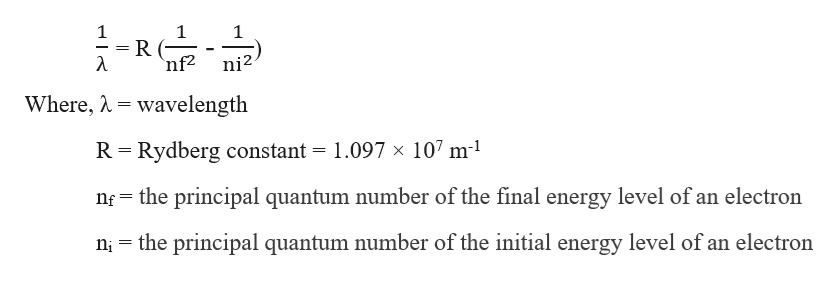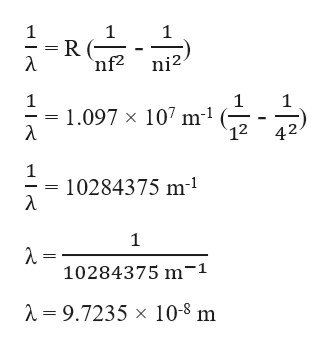# Hydrogen atoms are excited by a laser to the ?=4 state and then allowed to emit.What is the maximum number of distinct emission spectral lines (lines of different wavelengths) that can be observed from this system?57284316Calculate the wavelength of the 4⟶1 transition.?=__________m

Question
86 views

Hydrogen atoms are excited by a laser to the ?=4 state and then allowed to emit.

What is the maximum number of distinct emission spectral lines (lines of different wavelengths) that can be observed from this system?

5

7

2

8

4

3

1

6

Calculate the wavelength of the 4⟶1 transition.

?=__________m

check_circle

Step 1

Given, n = 4

The maximum number of distinct emission spectral lines (lines of different wavelengths) that can be observed from this system can be calculated as:

Step 2

The Rydberg equation for the calculation of the wavelength of an atomic element when an excited electron moves from the quantum shells is expressed as:help_outlineImage Transcriptionclose1 nf2 λ ni2 Where, wavelength R Rydberg constant = 1.097 x 107 m1 nf the principal quantum number of the final energy level of an electron the principal quantum number of the initial energy level of an electron ni fullscreen
Step 3

Given, 4⟶1 transition

nf = 1

ni = 4

Therefore, wavelength of the spec...help_outlineImage Transcriptionclose1 1 = R ( 'nf2 1 ni2 1 1.097 x 107 m1 λ 12 42 1 10284375 m1 λ 1 λ- 10284375 m-1 =9.7235 x 10-8 m fullscreen

### Want to see the full answer?

See Solution

#### Want to see this answer and more?

Solutions are written by subject experts who are available 24/7. Questions are typically answered within 1 hour.*

See Solution
*Response times may vary by subject and question.
Tagged in

### General Chemistry